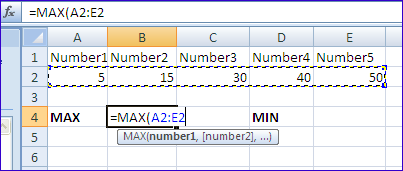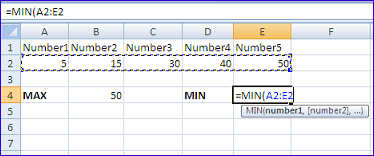# Learn Practical Use of MAX Function and MIN Function in Excel Worksheets

By | February 14, 2014

### What is MAX Function?Learn Practical Use of MAX Function and MIN Function in Excel Worksheets

The MAX function is used to get the maximum number from different given numbers in Excel Worksheet.

#### Syntax of MAX Function in Excel

MAX(number1,number2,number3,…numberN)
For example the function MAX(5,100,35) will return 100, since 100 is largest number of all 5, 100 and 35.

### Syntax of MAX Function using Cell References

MAX(CellRef1:CellRef2)
Where CellRef1 is the cell reference of the first cell and CellRef2 is the cell reference of the last cell in the given cell range in Excel Worksheet.

### What is MIN Function?The MIN function is used to get the minimum number from different given numbers in Excel Worksheet.

#### Syntax of MIN Function in Excel

MIN(number1,number2,number3,…numberN)
For example the function MIN(5,100,35) will return 5, since 5 is the smallest number of all 5, 100 and 35.

### Syntax of MIN Function using Cell References

MIN(CellRef1:CellRef2)
Where CellRef1 is the cell reference of the first cell and CellRef2 is the cell reference of the last cell in the given cell range in Excel Worksheet.

### How To Implement MAX Function in Excel

First of all place the cursor in an empty cell to enter the function.

• Type =
• Type the function name MAX
• Type the left parenthesis (
• Select the cell range A2 to E2 with the help of mouse so that the arguments A2:E2 will be automatically entered in the MAX function.
• Note: that you may type A2:E2 by keyboard also.
• Press Enter key (You need not to enter right parenthesis, it will be automatically included)
• The result 50 will be displayed in the cell.

### How To Implement MIN Function in Excel

First of all place the cursor in an empty cell to enter the function.

• Type =
• Type the function name MIN
• Type the left parenthesis (
• Select the cell range A2 to E2 with the help of mouse so that the arguments A2:E2 will be automatically entered in the MIN function.
• Note: that you may type A2:E2 by keyboard also.
• Press Enter key (You need not to enter right parenthesis, it will be automatically included)
• The result 5 will be displayed in the cell.

### Example Worksheet Picture For MAX and MIN FunctionsPractical Use of Max Function and MIN Min Function in Excel Worksheet